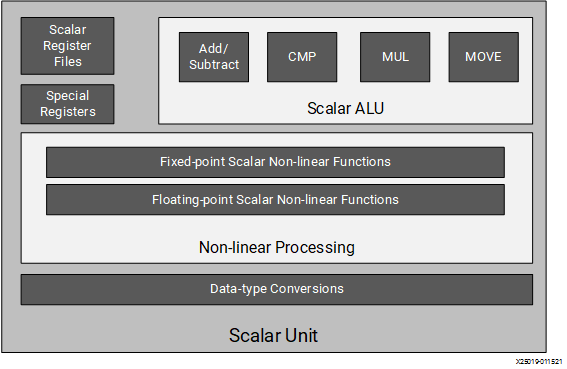# Scalar Processing Unit - 2022.1 English

## AI Engine Kernel Coding Best Practices Guide (UG1079)

Document ID
UG1079
Release Date
2022-05-25
Version
2022.1 English

The following figure shows the sub-components of the scalar unit. The scalar unit is used for program control (branch, comparison), scalar math operations, non-linear functions, and data type conversions much like a general-purpose processor. Similar to a general-purpose processor, generic C/C++ code can be used.

Figure 1. Scalar Processing UnitThe register files are used to store input and output. There are dedicated registers for pointer arithmetic, as well as for general-purpose usage and configuration. Special registers include stack pointers, circular buffers, and zero overhead loops. Two types of scalar elementary non-linear functions are supported in the AI Engine, fixed-point and floating-point precisions.

Fixed-point, non-linear functions include:

• Sine and cosine
• Absolute value (ABS)
• Comparison to find minimum or maximum (lesser than (LG)/greater than (GT))
• Square root
• Inverse square root and inverse

Floating-point, non-linear functions include:

• Square root
• Inverse square root
• Inverse
• Absolute value (ABS)
• Comparison to find minimum or maximum (lesser than (LG)/greater than (GT))

The arithmetic logic unit (ALU) in the AI Engine manages the following operations with an issue rate of one instruction per cycle.

• Integer addition and subtraction of 32 bits. The operation has a one-cycle latency.
• Bit-wise logical operation on 32-bit integer numbers (BAND, BOR, and BXOR). The operation has a one-cycle latency.
• Integer multiplication: 32 x 32-bit with an output result of 32 bits stored in the R register file. The operation has a three-cycle latency.
Note: Integer result is truncated to 32 bits when overflow occurs.
• Shift operation: Both left and right shift are supported. The operation has a one-cycle latency.
Note: A multiplication by a power of two is by default reduced to a shift operation.

Data type conversion can be done using `aie::to_fixed` and `aie::to_float`. This conversion can also support sqrt, inv, and inv_sqrt fixed-point operations.

## Scalar Programming

The compiler and scalar unit provide the programmer the ability to use standard ‘C’ data types. The following table shows standard C data types with their precisions. All types except float and double support signed and unsigned prefixes.

Table 1. Scalar data types
Data Type Precision Comment
char 8-bit signed
short 16-bit signed
int 32-bit signed Native support
long 64-bit signed
float 32-bit
double 64-bit Emulated using softfloat library. Scalar proc does not contain FPU.

It is important to remember that control flow statements such as branching are still handled by the scalar unit even in the presence of vector instructions. This concept is critical to maximizing the performance of the AI Engine.